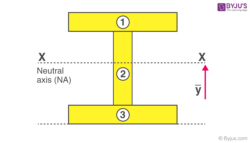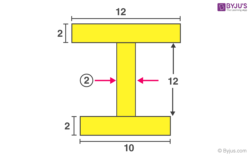# Moment of Inertia of i Section

Moment of Inertia is the quantity that expresses an object’s resistance to change its angular acceleration. It is a measure of rotational inertia of the body. To calculate the moment of inertia of i section the value of the centre of mass of the i section should be known. The value of the area, the moment of inertia of individual segments and the vertical distance from the centroid of the segment can be calculated using the data given in the problem.

## How to Find Moment of Inertia of “I” Section

The following steps should be followed to find the moment of inertia of the I section.

Step 1: The beam sections should be segmented into parts

The I beam section should be divided into smaller sections. The moment of inertia must be calculated for the smaller segments. Here, the section is divided into three rectangular segments.Step 2: Mark the neutral axis

The neutral axis is the horizontal line passing through the centre of mass. The centre of mass is calculated using the following formula

$\bar{y}$ = ΣYiAi/ΣAi

Yi is the centre of mass of the individual rectangle

Ai is the area of the individual rectangle

Step 3: Calculating the Moment of Inertia

Using the parallel axis theorem the total momentum is calculated as

$I_{total}=\sum (\bar{I_{i}}+A_{i}d_{i}^{2})$

where,

$(\bar{I_{i}})$ is the inertia of the individual segment about its own centroid axis.

Ai is the individual segment

di is the vertical distance from the centroid of the segment.

The moment of inertia of each of the rectangular parts must be calculated. The moment of inertia equation of a rectangle about its centroid axis is

$\bar{I} = \frac{1}{12}bh^{3}$

Where,

b is the base of the rectangle

h is the height of the rectangle

## ExampleLet us understand the above equation with an example

Finding the centre of mass of the I section

$\bar{y}$ = ΣYiAi/ΣAi

={[(2 + 12 + (2/2))(12 x 2)] + [2+ (12/2)(12 x2)]} + [(2/2)(10 x2)/ {20 + 24 x+24}

= {15(24) + 8(24) + 1(20)}/(20 + 24 +24)

= 360 + 192 + 20/(20 + 24 + 24)

= 572/68

= 8.4

Centre of mass of the I section is given at 4 mm

Segment 1: Upper rectangle

$(\bar{I_{1}})$ = (1/12)(12)(2)3

= 8

A1 = (12 x 2) = 24

$d_{1}= \left | y_{1}-\bar{y} \right |$

= |(2+12+{2/2}) – 8.4 | = 6.6

($(\bar{I_{1}})$ + A1d12) = (8 + 24(6.6)2) = (8 + 1045) = 1053

Segment 2: Middle rectangle

$(\bar{I_{2}})$ = (1/12)(12)(2)3

= 8

A2 = (12 x 2) = 24

$d_{2} = \left | y_{2}-\bar{y} \right |$

= |(2+ (12/2) – 8.4|= (8 – 8.4) = 0.4

($(\bar{I_{2}})$ + A2d22) = (8 + 24(0.4)2) =11.84

Segment 3: Lower rectangle

$(\bar{I_{3}})$ = (1/12)(10)(2)3

= 6.66

A2 = (10 x 2) = 20

$d_{3} = \left | y_{3}-\bar{y} \right |$

= |((2/2)- 8.4)|= 7.4

($(\bar{I_{3}})$ + A1d12) = (6.66 + (20)(7.4)2)= 1101.9

$I_{total}=\sum (\bar{I_{i}}+A_{i}d_{i}^{2})$

= ($(\bar{I_{1}})$ + A1d12)+ ($(\bar{I_{2}})$ + A2d22)+ ($(\bar{I_{3}})$ + A1d12) =1053 + 11.84

+ 1101.9 = 2166 mm4

Moment of inertia of T section, Itotal = 2166 mm4